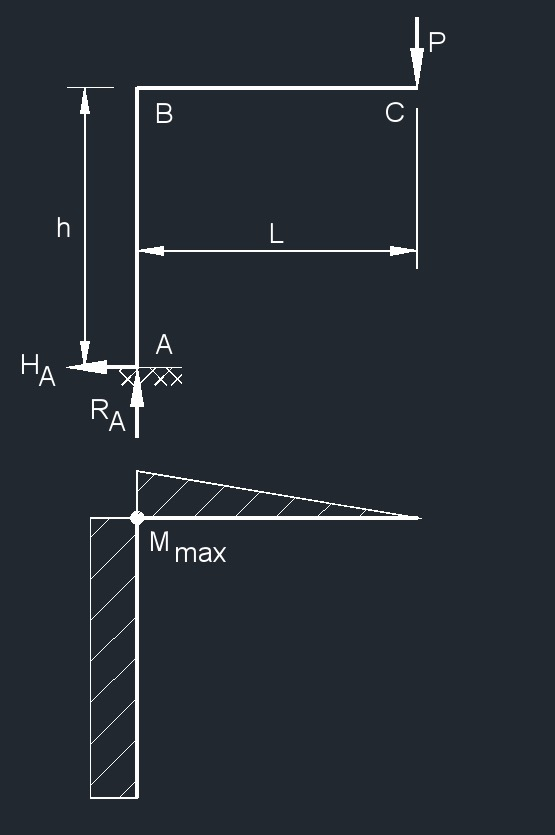# Two Member Frame - Fixed/Free Free End Vertical Point Load

Written by Jerry Ratzlaff on . Posted in Structural

##formulas that use Two Member Frame - Fixed/Free Free End Vertical Point Load

 $$\large{ R_A = P }$$ $$\large{ H_A = 0 }$$ $$\large{ M_{max} \left(at \;points\; A\; and \;B\right) = P\;L }$$ $$\large{ \Delta_{Cx} = \frac{P\;L\;h^2}{2\; \lambda \; I} }$$ $$\large{ \Delta_{Cy} = \frac{P\;L^2}{3\; \lambda \; I} \; \left( L + 3\;h \right) }$$ $$\large{ \theta_{C} = \frac{P\;L}{2\; \lambda \; I} \; \left( L + 2\;h \right) }$$

Where:

$$\large{ \Delta }$$ = deflection or deformation

$$\large{ h }$$ = height of frame

$$\large{ H }$$ =  horizontal reaction load at bearing point

$$\large{ M }$$ = maximum bending moment

$$\large{ \lambda }$$  (Greek symbol lambda) = modulus of elasticity

$$\large{ A, B, C }$$ = points of intersection on frame

$$\large{ R }$$ = reaction load at bearing point

$$\large{ I }$$ = second moment of area (moment of inertia)

$$\large{ \theta }$$  (Greek symbol theta) = slope of member

$$\large{ L }$$ = span length of the bending member

$$\large{ P }$$ = total concentrated load